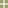Chi-square TestsMain Concepts | Demonstration  | Activity  | Teaching Tips  | Data Collection & Analysis  | Practice Questions  | Milestone   | Fathom TutorialMain concepts • The tests in this unit are different from the earlier ones in several ways. First of all, the focus is not on estimating a parameter, so there will be nothing about confidence intervals here. Second, the distribution family we'll be using for our sampling distribution is not symmetric and bell-shaped like the normal and t distributions. Third, the data we'll be looking at are strictly categorical. •We have seen several statistics that all had approximately t distributions. Similarly, in this unit we'll look at three contexts in which the preferred test statistic has a chi-squared distribution. These tests are not the same even though they have the same name (chi-square) and approximately the same distribution. These three tests are the Test of Independence, the Test of Homogeneity and the Goodness of Fit Test. Keep them distinct. • The "goodness-of-fit test" is a way of determining whether a set of categorical data came from a claimed discrete distribution or not. The null hypothesis is that they did and the alternate hypothesis is that they didn't. It answers the question: are the frequencies I observe for my categorical variable consistent with my theory? The goodness-of-fit test expands the one-proportion z-test. The one-proportion z-test is used if the outcome has only two categories. The goodness-of-fit test is used if you have two or more categories. • The "test of homogeneity" is a way of determining whether two or more sub-groups of a population share the same distribution of a single categorical variable. For example, do people of different races have the same proportion of smokers to non-smokers, or do different education levels have different proportions of Democrats, Republicans, and Independent. The test of homogeneity expands on the two-proportion z-test. The two proportion z-test is used when the response variable has only two categories as outcomes and we are comparing two groups. The homogeneity test is used if the response variable has several outcome categories, and we wish to compare two or more groups. • The "test of independence" is a way of determining whether two categorical variables are associated with one another in the population, like race and smoking, or education level and political affiliation. In the probability unit we looked at this question without paying attention to the variability of our sample. Now we will have a method for deciding whether our observed P(A|B) is "too far" from our observed P(A) to conclude independence. • If you're thinking, "homogeneity and independence sound the same!", you're nearly right. The difference is a matter of design. In the test of independence, observational units are collected at random from a population and two categorical variables are observed for each unit. In the test of homogeneity, the data are collected by randomly sampling from each sub-group separately. (Say, 100 blacks, 100 whites, 100 American Indians, and so on.) The null hypothesis is that each sub-group shares the same distribution of another categorical variable. (Say, "chain smoker", "occasional smoker", "non-smoker".) The difference between these two tests is subtle yet important. • Note that in the test of independence, two variables are observed for each observational unit. In the goodness-of-fit test there is only one observed variable. • As with all other tests, certain conditions must be checked before a chi-square test of anything is carried out. See the Teaching Tips for more on this.